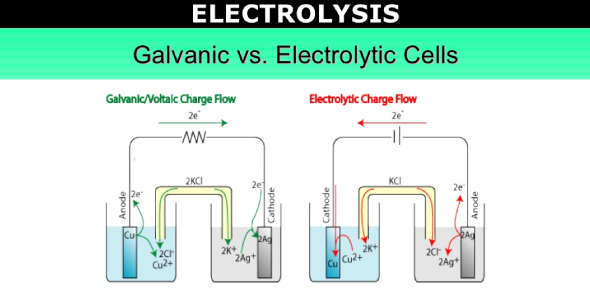# Electrolysis And Electrolytic Cell Test! Quiz

15 Questions | Attempts: 2181
ShareSettingsDo you know anything about Electrolysis and Electrolytic cells? Do you think you can pass this quiz? An electrolytic cell utilizes electrical energy to drive a non- spontaneous redox reaction. The cell is often used to decompose chemical compounds in a process called electrolysis. It is a technique that uses a direct electric current. Take this quiz and learn more about electrolysis and electrolytic cells.

• 1.
The use of electric energy to bring about a chemical reaction is ____________.
• A.

A) corrosion

• B.

B) spontaneous combustion

• C.

C) a galvanic cell

• D.

D) an electrolytic ell

• 2.
In an electrolytic cell, the negative electrode is called the __________.
• A.

A) anode, at which oxidation occurs

• B.

B) anode, at which reduction occurs

• C.

C) cathode, at which oxidation occurs

• D.

D) cathode, at which reduction occurs

• 3.
Which of the following statements is INCORRECT?
• A.

A) In an electrolytic cell, reduction occurs at the anode.

• B.

B) Aluminum metal would form at the cathode during the electrolysis of molten AlBr3.

• C.

C) The cathode is labeled "+" in an electrolytic cell.

• D.

D) Electrons flow from the anode to the cathode in electrolytic cells.

• 4.
__________ are electrical conductors which are placed in an electrolyte to provide surfaces for oxidation and reduction half-reactions.
• A.

A) Electrolytes

• B.

B) Electrodes

• C.

C) Ions

• D.

D) Electrolysis

• 5.
The anode and cathode are determined by the __________.
• A.

A) site of oxidation and reduction half-reactions.

• B.

B) movement of ions in the electrolyte

• C.

C) connection of terminals of the external sources

• D.

D) reduction potential s of ions

• 6.
Which of the following pair is CORRECT?
• A.

A) Anode: positive terminal where reducation occurs; Cathode: negative terminal where oxidation occurs

• B.

B) Anode: negative terminal where oxidation occurs; Cathode: postive terminal where reduction occurs

• C.

C) Anode: negative terminal where reduction occurs; Cathode: postive terminal where oxidation occurs

• D.

D) Anode: positive terminal where oxidation occurs; Cathode: negative terminal where reduction occurs

• 7.
Which of the following statement about the transfer of charge in an electrolytic cell is        INCORRECT?
• A.

A) Negative ions are called anions and positive ions are called cations.

• B.

B) Anions move through the electrolyte to the cathode and cations move to the anode.

• C.

C) An electrolyte conducts electricity by the movement of dissolved anions and cations.

• D.

D) Electrons travel from the negative terminal of the source to the cathode, and from the anode to the positive terminal of the source.

• 8.
Which of the following is NOT an electrolyte?
• A.

A) Sodium chloride solution

• B.

B) Aqueous ammonia

• C.

C) Molten sodium chloride

• D.

D) Molten naphtalene

• 9.
During the electrolysis of molten sodium iodide, sodium ions move ________.
• A.

A) to the anode, which is positively charged

• B.

B) to the cathode, which is positively charged

• C.

C) to the cathode, which is negatively charged

• D.

D) to the anode, which is negatively charged

• 10.
The diagram above shows the electrolysis of molten lead(II) bromide, PbBr2 using platinum electrodes. An electric current flows through molten lead (II) bromide for 10 minutes. What can be observed at the anode of the platinum electrode?
• A.

A) A brown gas is released.

• B.

B) A grey solid is deposited.

• C.

C) The platinum electrode is corroded.

• D.

D) No observation.

• 11.
What can be observed at the cathode of the platinum electrode?
• A.

A) A brown gas is released.

• B.

B) A grey solid is deposited.

• C.

C) The platinum electrode is corroded.

• D.

D) No observation.

• 12.
Which of the following are correct half-equations for the reactions happen at the anode and cathode?
• A.

A) Anode: 2Br-(l) --> Br2(g) + 2e-; Cathode: Pb(s) --> Pb2+(l) + 2e-

• B.

B) Anode: Pb2+(l) + 2e- --> Pb(s); Cathode: 2Br-(l) --> Br2(g) + 2e-

• C.

C) Anode: 2Br-(l) --> Br2(g) + 2e-; Cathode: Pb2+(l) + 2e- --> Pb(s)

• D.

D) Anode: Pb2+(l) + 2e- --> Pb (s); Cathode: Br2(g) + 2e- --> 2Br-(l)

• 13.
The electrolysis of an aqueous sodium chloride solution using inert electrodes produces gaseous chlorine at one electrode. At the other electrode, gaseous hydrogen is produced, and the solution around the electrode becomes basic. Which of the following equations is the correct equation for the cathode half-reaction in this electrolytic cell?
• A.

A) H2 + 2OH- → 2H2O + 2e-

• B.

B) 2H2O + 2e- → H2 + 2OH-

• C.

C) 2Cl- → Cl2 + 2e-

• D.

D) Cl2 + 2e- → 2Cl-

• 14.
Under standard conditions, which one of the following statements about the half cell process is true for the cell?
• A.

A) Cu2+ is reduced at the anode

• B.

B) Cu2+ is reduced at the cathode

• C.

C) Cd2+ is reduced at the anode

• D.

D) Cd2+ is reduced at the cathode

• 15.
Which of the following will NOT affect the selective discharge of ions?
• A.

A) Types of electodes

• B.

B) Types of electrolyte

• C.

C) Positions of ions in the eletrochemical series

• D.

D) Concentration of ions in electrolysis

## Related TopicsBack to top
×

Wait!
Here's an interesting quiz for you.